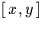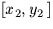Next: SLA_KBJ - Select Epoch Prefix
Up: SUBPROGRAM SPECIFICATIONS
Previous: SLA_INTIN - Decode an Integer Number

## SLA_INVF - Invert Linear Model

ACTION:
Invert a linear model of the type produced by the sla_FITXY routine.

CALL:
CALL sla_INVF (FWDS,BKWDS,J)

GIVEN:

 D(6) FWDS model coefficients

RETURNED:

 D(6) BKWDS inverse model J I status: 0 = OK, -1 = no inverse

NOTES:
1.
The models relate two sets ofcoordinates as follows. Naming the six elements of FWDS a,b,c,d,e & f, where two sets of coordinates [x1,y1] andare related thus:
x2 = a + bx1 + cy1
y2 = d + ex1 + fy1
The present routine generates a new set of coefficients p,q,r,s,t & u (the array BKWDS) such that:
x1 = p + qx2 + ry2
y1 = s + tx2 + uy2
2.
Two successive calls to this routine will deliver a set of coefficients equal to the starting values.
3.
To comply with the ANSI Fortran 77 standard, FWDS and BKWDS must not be the same array. The routine is, in fact, coded so as to work properly on the VAX and many other systems even if this rule is violated, something that is not, however, recommended.
4.